A sparse set is a simple data structure that has a few interesting properties:

• O(1) to add an item.

• O(1) to remove an item.

• O(1) to lookup an item.

• O(1) to clear the set.

• O(n) to iterate over the set.

• A set does not require its internal items storage to be initialised upon creation (!).

Sparse sets are commonly used to implement Entity Component System architectural pattern. I plan to cover ECS in one of my future posts but for now let’s try to understand and implement sparse sets.

## Overview

Sparse sets use two integer arrays internally: `dense` and `sparse`. The former is a packed array that stores the set’s items (integers) in the insertion order. The latter is an array that can have holes (hence the name – `sparse`) and it maps the set’s items to their indices in `dense`. The set also keeps track of how many items it has. We call it `N`.

Sparse sets could be implemented as growable, i.e. being able to reallocate the memory they use but I will use non-growable version in this post for simplicity. This means a user has to specify the size of the set upon creation.

``````/** An item stored in a sparse set. */
typedef uint32_t rho_ss_id;

/** Maximum possible value of the rho_ss_id. */
static const rho_ss_id rho_ss_id_max = UINT32_MAX - 1;

/** The sparse set. */
struct rho_ss {
rho_ss_id *sparse;  /**< Sparse array used to speed-optimise the set.     */
rho_ss_id *dense;   /**< Dense array that stores the set's items.         */
rho_ss_id  n;       /**< Number of items in the dense array.              */
rho_ss_id  max;     /**< Maximum allowed item ID.                         */
};``````

## An empty set

We start with an empty set that can have up to `max` items. The next picture demonstrates the sparse set that can hold up to ten items.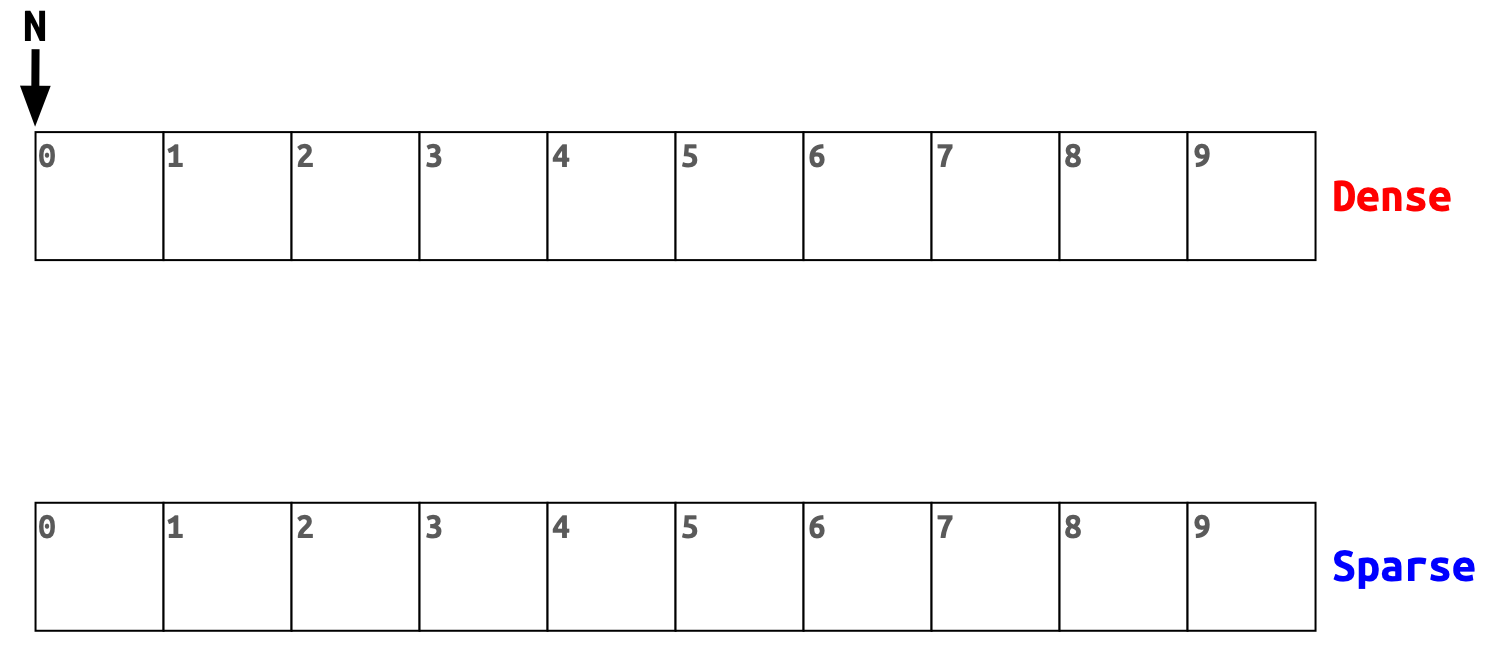`N` is zero, `dense` and `sparse` are allocated but not initialised.

``````struct rho_ss rho_ss_alloc( rho_ss_id max_id )
{
assert( max_id > 0 && max_id <= rho_ss_id_max );

size_t        array_size = sizeof( rho_ss_id ) * ( max_id + 1 );
struct rho_ss ss         = { 0 };

ss.dense  = malloc( array_size );
ss.sparse = malloc( array_size );

if ( !ss.dense || !ss.sparse ) {
free( ss.dense );
free( ss.sparse );

ss.dense  = NULL;
ss.sparse = NULL;
} else {
ss.max = max_id;
}

return ss;
}``````

Let’s add `4` as a first item to the set.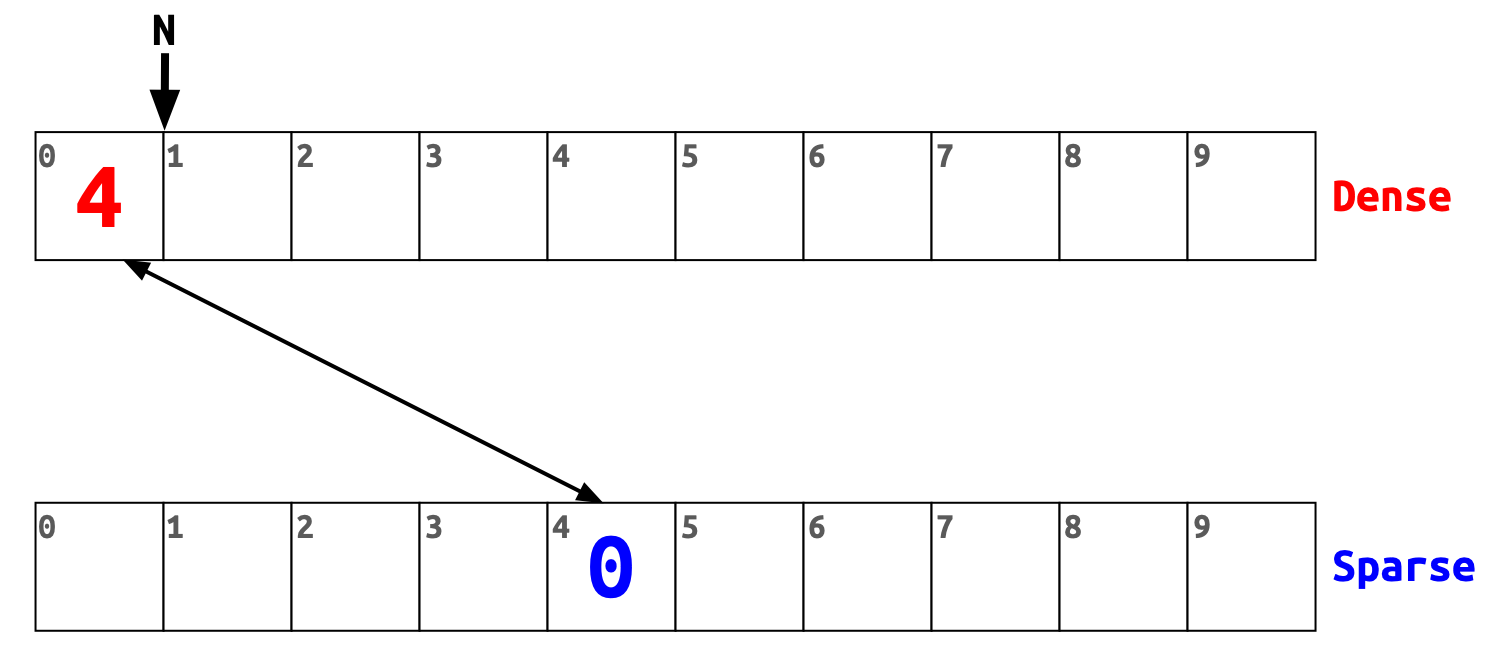First, we add the item to the `dense` at index `0` (this is the current value of `N`). Then we write `N` to the `sparse` array at index `4`. Now both slots in the `dense` and `sparse` arrays point to each other. Lastly, we increase `N` by one.

Let’s add `6` as a second item.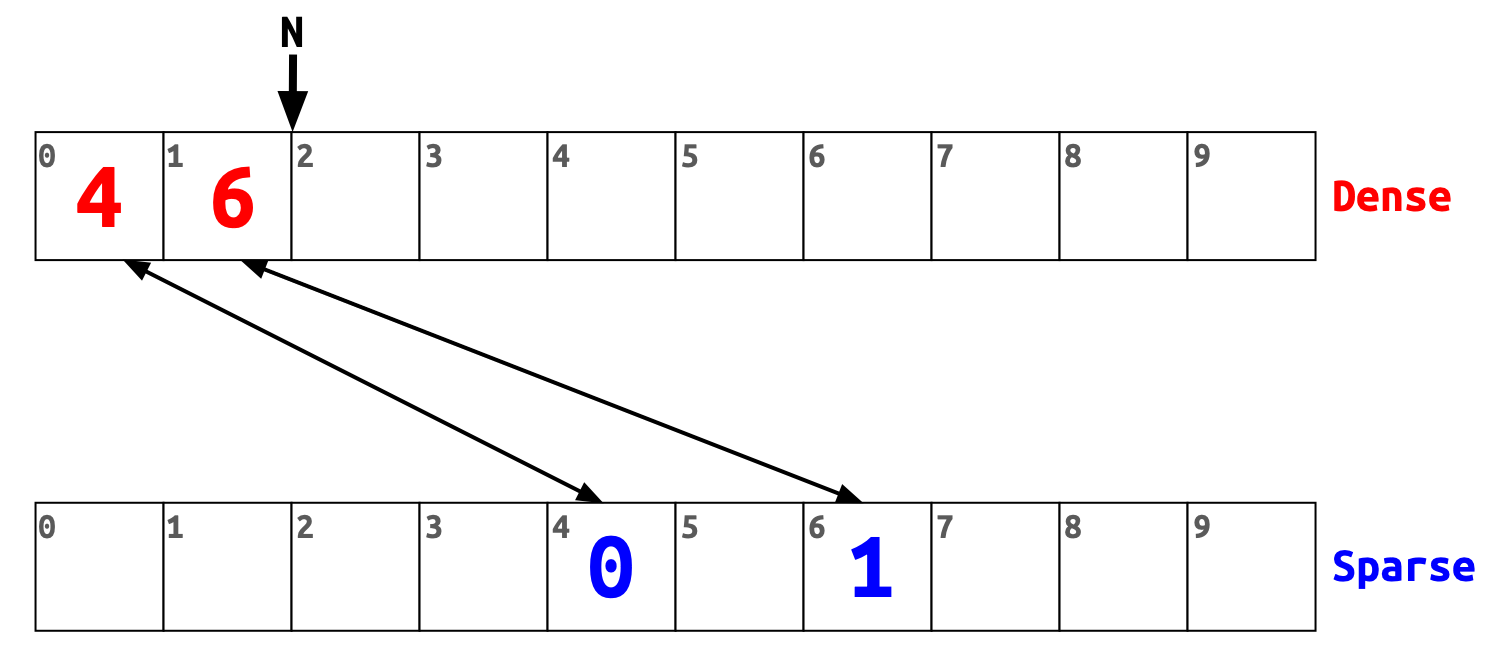The steps are the same. We put `6` to the next free slot of the `dense` array, write `N` to the `sparse` at index `6`, and increase `N` by one.

The `sparse` array has holes, while the `dense` array items are placed next to each other.

Now we add `0` as a third item.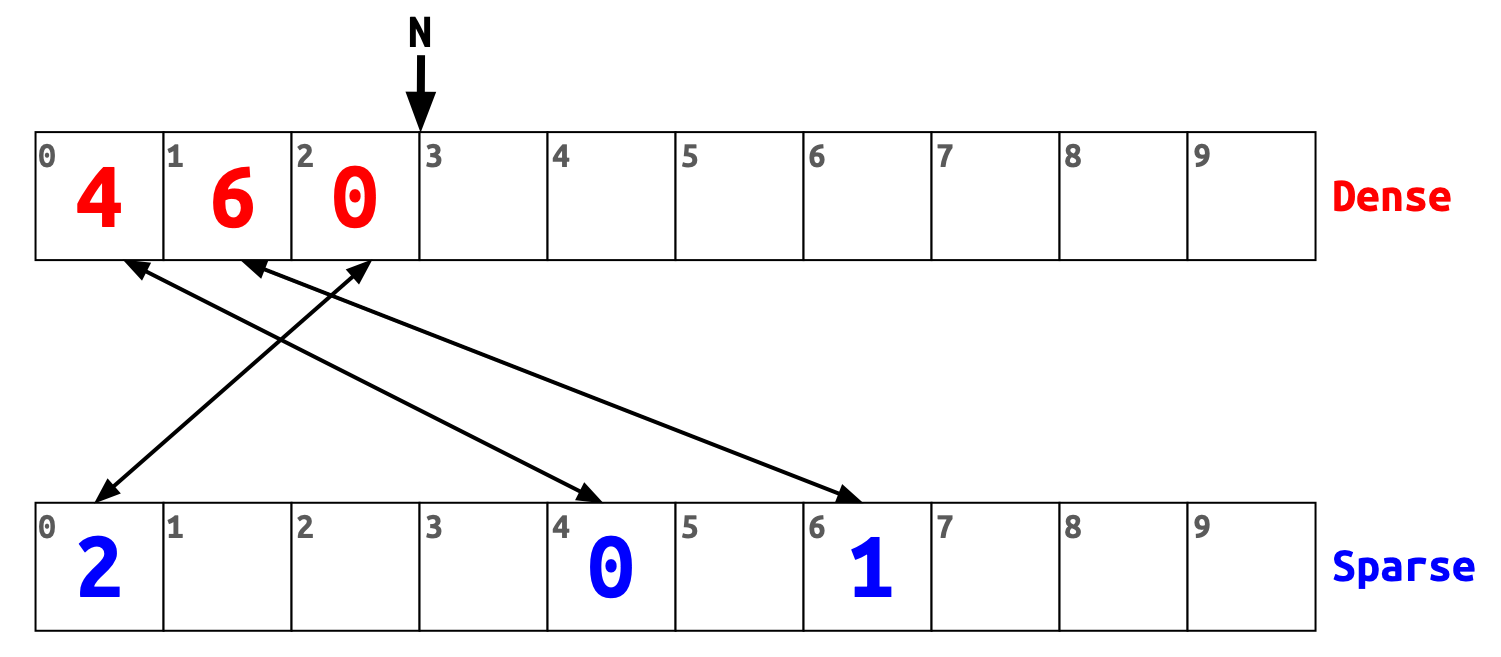There is nothing new here. The item we added is `0` so we put its `dense` index (which is `2`) to `sparse` at index `0`.

And this is how we can implement it:

``````void rho_ss_add( struct rho_ss *ss, rho_ss_id id )
{
assert( ss );
assert( id <= ss->max );

if ( rho_ss_has( ss, id ) ) {
return;
}

ss->dense[ss->n] = id;
ss->sparse[id]   = ss->n;

++ss->n;
}``````

The `rho_ss_has` function checks if the given item is in the sparse set. We implement it in the next section.

## Checking whether an item is in the set

Let’s test if `6` is in the set. To do so we go to the `sparse` array and check its value at index `6`. The value is `1`. Now we use this value as an index in the `dense` array. The `dense` has value `6` at this index, which means 'yes, the set contains item six'.

Now let’s check if `9` is in the set. We go to the `sparse` array and check its value at index `9`. The value is garbage (we haven’t initialised the memory, remember?) and can be anything, let’s say it is `X`.

If `X >= N` then `X` is out of bounds index for the `dense` array and `9` is not in the set.

Suppose `X` is less than `N`. In our case it should be either of `0`, `1`, or `2` because `N` is `3`. We check if the `dense` array’s value at index `X` is `9`. It is not and it cannot be, so the answer is 'no, the set does not contain item nine'.

Now we can implement the operation:

``````bool rho_ss_has( struct rho_ss *ss, rho_ss_id id )
{
assert( ss );
assert( id <= ss->max );

rho_ss_id dense_index = ss->sparse[id];

return dense_index < ss->n && ss->dense[dense_index] == id;
}``````

## Removing an item

Let’s remove `6` from the set.

First, we replace `6` with the last item from the `dense` array, which is `0`. Then we have to update the `spare` array at index `0` with the new index of `0` in the `dense` array, which is `1`. And then reduce `N` by one.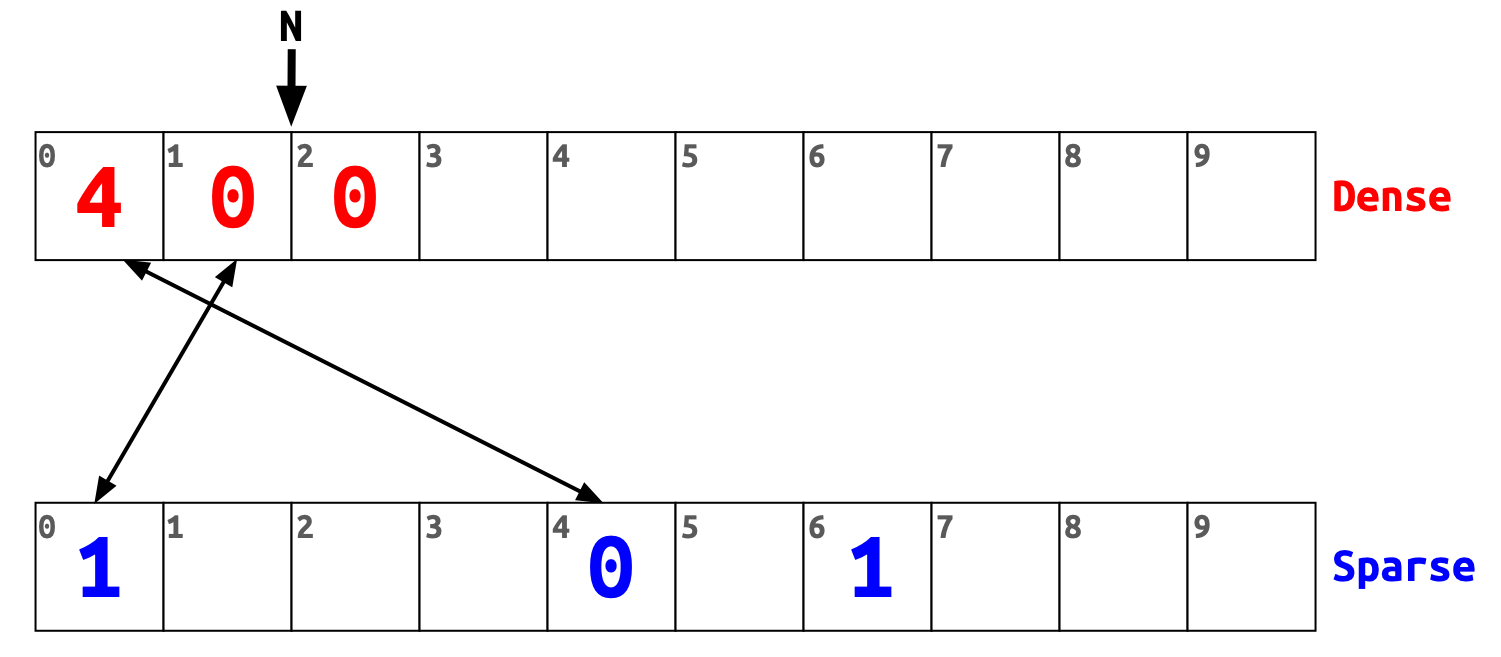In other words to remove an item from the set, we replace it with the last item in the `dense` array and update the corresponding `sparse` array’s slot to point to the new location.

The implementation is as follows:

``````void rho_ss_remove( struct rho_ss *ss, rho_ss_id id )
{
assert( ss );
assert( id <= ss->max );

if ( rho_ss_has( ss, id ) ) {
--ss->n;
rho_ss_id dense_index  = ss->sparse[id];
rho_ss_id item         = ss->dense[ss->n];
ss->dense[dense_index] = item;
ss->sparse[item]       = dense_index;
}
}``````

## Iterating over the set

This one is super simple. You just iterate over the `dense` array.

Like this (highlighted lines):

``````struct rho_ss ss = rho_ss_alloc( 10 );

for ( rho_ss_id i = 0; i < ss.n; ++i ) {
printf( "%d ", ss.dense[i] );
}
printf( "%s", "\n" );

rho_ss_free( &ss );``````

## Clearing the set

Just set `N` to zero:

``````void rho_ss_clear( struct rho_ss *ss )
{
assert( ss );

ss->n = 0;
}``````

Like I said before, most of the sparse set operations are super fast.

## What’s next?

You could play with my implementation of the sparse set or (and this is preffered way) implement it on your own.